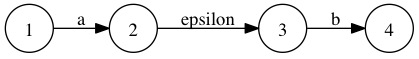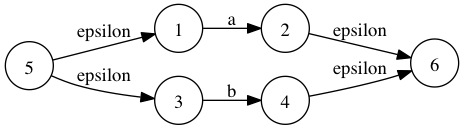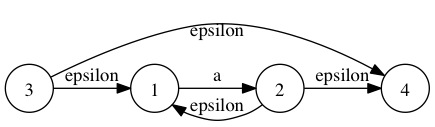``````concatenation: abc    表示匹配字符串abc
alternation: abc|def  表示匹配字符串abc或者def
closure: a*           表示匹配零个到多个a构成的字符串
``````

###正则语法的分析

``````re ::= alter
re_base ::= char | char_range | '(' re ')'
alter ::= alter_base alter_end
alter_base ::= concat
alter_end ::= '|' alter_base alter_end | epsilon
concat ::= concat_base concat_end
concat_base ::= re_base | closure
concat_end ::= concat_base concat_end | epsilon
closure ::= re_base '*'
``````

``````class ASTNode
{
public:
ACCEPT_VISITOR() = 0;
virtual ~ASTNode() { }
};
class CharNode : public ASTNode
{
public:
explicit CharNode(int c) : c_(c) { }
ACCEPT_VISITOR();
int c_;
};
class CharRangeNode : public ASTNode
{
public:
struct Range
{
int first_;
int last_;
explicit Range(int first = 0, int last = 0)
: first_(first), last_(last)
{
}
};
CharRangeNode() { }
void AddRange(int first, int last)
{
ranges_.push_back(Range(first, last));
}
void AddChar(int c)
{
chars_.push_back(c);
}
ACCEPT_VISITOR();
std::vector<Range> ranges_;
std::vector<int> chars_;
};
class ConcatenationNode : public ASTNode
{
public:
void AddNode(std::unique_ptr<ASTNode> node)
{
nodes_.push_back(std::move(node));
}
ACCEPT_VISITOR();
std::vector<std::unique_ptr<ASTNode>> nodes_;
};
class AlternationNode : public ASTNode
{
public:
void AddNode(std::unique_ptr<ASTNode> node)
{
nodes_.push_back(std::move(node));
}
ACCEPT_VISITOR();
std::vector<std::unique_ptr<ASTNode>> nodes_;
};
class ClosureNode : public ASTNode
{
public:
explicit ClosureNode(std::unique_ptr<ASTNode> node)
: node_(std::move(node))
{
}
ACCEPT_VISITOR();
std::unique_ptr<ASTNode> node_;
};
``````

###字符 (边) 集的构造

AST构造好了之后，需要把AST转换成NFA。语法中有[a-zA-Z0-9]这种字符区间表示法，我们可以用最简单原始的方法转换，就是把区间中的每个字符都转化成相应的一条边 (NFA中的边) ，这样一来会导致字符区间越大，对应边的数量会越多，使得对应的NFA也越大。因此，我们需要构造区间字符集合来减少边的数量。

 这需要构造一个字符集，每次插入一个区间的时候，把新插入的区间与已存在的区间进行分割，初始时已存在的区间集为空，那么正则表达式a[x-z] [a-z]*e的划分步骤如下：

``````class EdgeSetConstructorVisitor : public Visitor
{
public:
explicit EdgeSetConstructorVisitor(EdgeSet *edge_Setter)
: edge_Setter_(edge_Setter)
{
}
EdgeSetConstructorVisitor(const EdgeSetConstructorVisitor &) = delete;
void operator = (const EdgeSetConstructorVisitor &) = delete;

VISIT_NODE(CharNode);
VISIT_NODE(CharRangeNode);
VISIT_NODE(ConcatenationNode);
VISIT_NODE(AlternationNode);
VISIT_NODE(ClosureNode);

private:
EdgeSet *edge_Setter_;
};
``````

###生成epsilon-NFA

epsilon-NFA是包含epsilon边 (空边) 的NFA，把简单正则表达式转换成epsilon-NFA的方法如下：

• 正则表达式：ab 对应的epsilon-NFA是：
••  正则表达式：a b对应的epsilon-NFA是：
•• 正则表达式：a* 对应的epsilon-NFA是：
•``````a{m}       重复a，m次
a{m,n}     重复a，m到n次
a{m,}      重复a，至少m次
a+         重复a，至少1次
a?         重复a，0次或1次
``````

###epsilon-NFA subset construction to DFA

``````subset construction:
// 把start_state通过epsilon扩展得到起始subset
start_subset <- epsilon_extend(start_state)
// 初始化subsets
subsets <- { start_subset }
// 初始化work_list
work_list <- subsets
while (!work_list.empty())
{
subset <- work_list.pop_front()
// 取出NFA中的每条边
for edge in epsilon-NFA
{
// 对subset中的每个state通过edge所到达的
// state的epsilon边扩展得到next_subset
next_subset <- delta(subset, edge)

// 如果next_subset不存在于subsets中，
// 则把这个next_subset加入到work_list中
if (!subsets.exist(next_subset))
work_list.push_back(next_subset)

// 构建subset到next_subset的边映射
map[subset, edge] = next_subset

// 把next_subset合并到subsets
subsets.merge({next_subset})
}
}

delta:
// 初始化next_subset为空集合
next_subset <- { }
for state in subset
{
// 取出next_state并将它通过epsilon
// 边扩展得到的subset合并到next_subset中
next_state <- map[state, edge]
if (next_state)
next_subset.merge(epsilon_extend(next_state))
}
``````

``````epsilon_extend(1) –> { 1 }
epsilon_extend(2) –> { 1, 2, 4 }
epsilon_extend(3) –> { 1, 3, 4 }
epsilon_extend(4) –> { 4 }
``````

``````epsilon_extend_construct:
work_list <- { }
// 为每个state初始化epsilon_extend集合
for state in epsilon-NFA
{
epsilon_extend(state) <- { state }
work_list.push_back(state)
}
while (!work_list.empty())
{
state <- work_list.pop_front()
state_epsilon_extend <- epsilon_extend(state)
// 把state通过epsilon所能到达的state的epsilon_extend
// 合并到当前state的epsilon_extend
for next_state in map[state, epsilon]
state_epsilon_extend.merge(epsilon_extend(next_state))
// 如果当前state的epsilon_extend变化了之后
// 把所有通过边epsilon到达state的pre_state都加入到work_list中
if (state_epsilon_extend.has_changed())
{
for pre_state in epsilon_pre(state)
work_list.push_back(pre_state)
}
}
``````

epsilon-NFA通过subset construction构造成完之后，并把构造的subsets中的subset转换成DFA中的state，再把NFA中除epsilon边之外的所有边都转换成DFA的边，这样就把DFA构造完成。

###DFA minimization

``````minimization:
// 把所有state划分成accept的state集合和非accept的state集合
state_sets <- { {accept_state(DFA)}, {non_accept_state(DFA)} }
do
{
work_list <- state_sets
old_state_sets_size <- state_sets.size()
state_sets <- { }
for state_Setter in work_list
{
split_success <- false
for edge in DFA
{
// 如果edge可以把state_set拆分成两个subset，那就把新拆分出来的
// 两个subset合并到state_sets里面，并break继续work_list中取出下一个
// state_set拆分
subset1, subset2, split_success <- split(state_Setter, edge)
if (split_success)
{
state_sets.merge({subset1, subset2})
break
}
}
if (!split_success)
state_sets.merge({state_Setter})
}
} while (old_state_sets_size != state_sets.size())
``````

###正则引擎常见的实现方法

 DFA是三种实现中效率最高的，不过缺点也明显，一是DFA的构造复杂耗时，二是DFA支持的正则语法有限。在早期正则被发明出来时，只有Concatenation、Alternation、Kleene star，即ab a b a*，DFA可以轻松搞定。随着计算机的发展，正则像所有其它语言一样发展出各种新的语法，很多语法在DFA中难以实现，比如capture、backreference (capture倒是有论文描述可以在DFA中实现) 。

Backtracking是三种实现中效率最低的，功能确是最强的，它可以实现所有后面新加的语法，因此，大多数正则引擎实现都采用此方法。因为它是回溯的，所以在某些情况下会出现指数复杂度，这篇文章有详细的描述。

NFA(Thompson NFA)有相对DFA来说的构造简单，并兼有接近DFA的效率，并且在面对Backtracking出现指数复杂度时的正则表达式保持良好的性能。

###NFA-based的实现

 这里描述的NFA是指Thompson NFA。Thompson NFA实现的核心是对于正则表达式多个可能的匹配并发的向前匹配，此过程是在模拟DFA运行。比如对于正则表达式abab abbb匹配字符串abbb：

Backtracking的匹配过程是取正则的第一个子表达式abab匹配，前两个字符匹配成功，匹配第三个字符的时候失败，这时引擎回溯选择第二个子表达式abbb匹配，最终匹配成功。

Thompson NFA是同时取两个子表达式abab和abbb匹配，前两个字符匹配时，两个子表达式都能匹配成功，当匹配第三个字符时，子表达式abab匹配失败，因此丢弃，abbb匹配成功接着匹配，最终匹配成功。

repeat是一种会分化的状态，达到最小匹配次数时，可以接着往下走，也可以继续重复匹配repeat的子正则表达式，这样就分化成两条线了，并且每条线都带有自己的状态数据，因此，我的实现中引入的thread来表示一条匹配线，里面有状态数据。

###Match和Search

``````// |-----|--------|---------------|-----|-------------|
// | any | repeat | capture begin | ... | capture end |
// |-----|--------|---------------|-----|-------------|
``````

###结语